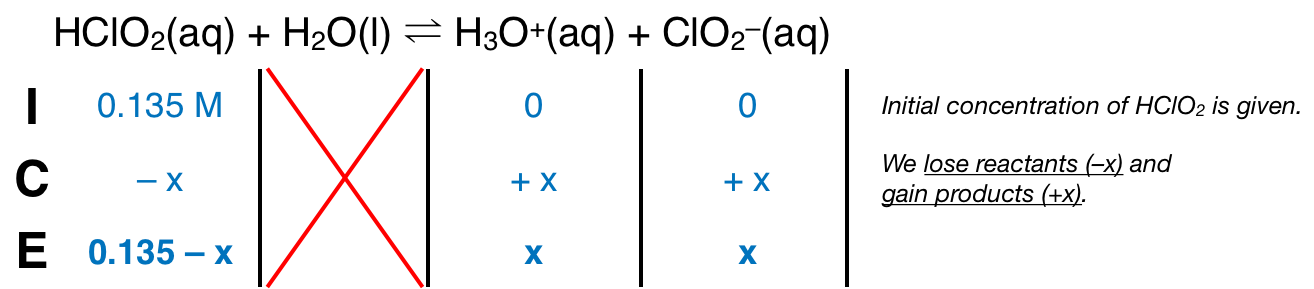# Problem: Find the pH of a 0.135 M aqueous solution of HCIO 2 , for which Ka = 1.1 x 10–2 .A) 1.25B) 3.28C) 1.17D) 1.34E) 1.48

###### FREE Expert Solution
88% (120 ratings)
###### FREE Expert Solution

We’re being asked to calculate the pH of a 0.135 M aqueous solution of HClO2.

Since HClO2 has a low Ka value, it’s a weak acid. Remember that weak acids partially dissociate in water and that acids donate H+ to the base (water in this case). The dissociation of HClO2 is as follows:

HClO2(aq) + H2O(l)  H3O+(aq) + ClO2(aq); Ka = 1.1 × 10–2

From this, we can construct an ICE table. Remember that liquids are ignored in the ICE table.The Ka expression for HClO2 is:Note that each concentration is raised by the stoichiometric coefficient: [HClO2], [H3O+] and [ClO2] are raised to 1.

88% (120 ratings)###### Problem Details

Find the pH of a 0.135 M aqueous solution of HCIO 2 , for which Ka = 1.1 x 10–2 .

A) 1.25

B) 3.28

C) 1.17

D) 1.34

E) 1.48

What scientific concept do you need to know in order to solve this problem?

Our tutors have indicated that to solve this problem you will need to apply the Weak Acids concept. If you need more Weak Acids practice, you can also practice Weak Acids practice problems.

What is the difficulty of this problem?

Our tutors rated the difficulty ofFind the pH of a 0.135 M aqueous solution of HCIO 2 , for wh...as high difficulty.

How long does this problem take to solve?

Our expert Chemistry tutor, Dasha took 12 minutes and 11 seconds to solve this problem. You can follow their steps in the video explanation above.

What professor is this problem relevant for?

Based on our data, we think this problem is relevant for Professor Walsby's class at SFU.